Teoria y Estructura Atómica

# Atomic Theory IV: Quantum Numbers and Orbitals

por Adrian Dingle, B.Sc., Anthony Carpi, Ph.D.

In Atomic Theory III: Wave-Particle Duality and the Electron, we discussed the advances that were made by Schrödinger, Born, Pauli, and others in the application of the quantum model to atomic theory. The Schrödinger equation was seen as a key mathematical link between the theory and the application of the quantum model. Born took the wave functions that Schrödinger produced and said that the solutions to the equation could define the energies and the most probable positions of electrons within atoms, thus allowing us to build a much more detailed description of where electrons might be found within an atom. This module further explores these solutions, the position of electrons, the shape of atomic orbitals, and the implications of these ideas.

## Waves and measurement uncertainty

As we saw in earlier reading, the electron is not a true particle, but a wave-particle similar to the photon. Since we measure the position of objects with light, the small size of the electron introduces a challenge. If we shine a light beam on a moving tennis ball, the light has little effect on the tennis ball and we can measure both its position and momentum with a high degree of accuracy. However, the electron is so tiny that even a single photon will influence its trajectory – thus if we shine a beam of light on it to measure its position, the energy of the photon will affect its momentum, and vice versa. Werner Heisenberg developed a principle to describe this uncertainty, called appropriately the Heisenberg Uncertainty Principle (Heisenberg, 1927). It tells us that mathematically, the product of the uncertainty in position (Δx) and the uncertainty in momentum (Δp) of an electron cannot be less than the reduced Planck constant ℏ/2 (Equation 1).Equation 1: The Heisenberg Uncertainty Principle equation, where Δx is the product of the uncertainty in position, Δp is the uncertainty in momentum of an electron, and ℏ/2 is the reduced Planck constant. (Equation created with CodeCogs online tool.)

This is a very small number that can usually be ignored, but when dealing with a particle as small as an electron, it is significant. Because of this, it becomes necessary to describe the position of an electron in terms of probability, rather than that of absolute certainty. We have to say that an electron is likely to be found within the atom in certain areas of high probability, but we cannot be 100% sure of its precise position.

Punto de Comprensión

The Heisenberg Uncertainty Principle tells us that

## Schrödinger solves the probability question

Because the electron is not a true particle, we cannot describe its movement or location in traditional terms. In other words, those that would normally be defined by simple x, y, and z coordinates. The challenges raised by the combination of wave particle duality and the Heisenberg Uncertainty Principle are simplified and expressed by Schrödinger’s equation that, when solved, produces wave functions denoted by Ψ. When Ψ is squared, the resulting solution gives the probability of finding an electron at a particular place in the atom. The Ψ2 term describes how electron density and electron probability are distributed in space around the nucleus of an atom.

### The simplest case - a hydrogen atom with a single electron

The application of the Schrödinger equation is most easily understood in the case of the hydrogen atom because the one-electron atom allows us to avoid the complex interactions of multiple electrons. The time-independent form of the Schrödinger equation (Equation 2) can be solved to provide solutions that correspond to the energy levels in a hydrogen atom. In solving this equation, we find that there are multiple solutions for Ψ that we call Ψ1, Ψ2, Ψ3, etc.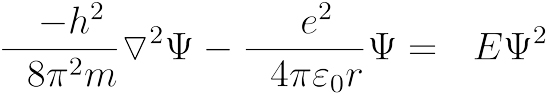Equation 2: The time independent Schrödinger equation for hydrogen's energy levels. (Equation created with CodeCogs online tool.)

Each of these solutions has a different energy that corresponds to what we think of as different energy levels, or electron shells, within the atom. The lowest energy electron distribution is that which is closest to the nucleus, and it is called the ground state. The ground state is the most stable state of the single electron within the hydrogen atom. Other, higher energy states exist and are called excited states. As the energy of each level increases above the ground state, we refer them to them as the second, third, fourth, etc. energy levels, respectively.

Punto de Comprensión

The energy of the electron shells ______ as you move away from the nucleus of the atom.

## Quantum numbers

Acceptable solutions to the wave equation for an electron cannot be just any values, but rather they are restricted to those that obey certain parameters. Those parameters are described by a set of three quantum numbers that are named the principal quantum number, the azimuthal quantum number, and the magnetic quantum number. These quantum numbers are given the symbols n, l, and m, respectively.

The principal quantum number n is a positive integer starting with a value of 1, where 1 corresponds to the first (lowest energy) shell known as the ground state in hydrogen. The principal quantum number then increases with the increasing energy of the shells (the excited states in hydrogen) to values of n = 2, n = 3, n = 4, etc., as one moves farther away from the nucleus to higher energy levels.

The azimuthal quantum number l (also called the orbital angular momentum quantum number) determines the physical, three-dimensional shape of the orbitals in any subshell. The value of l is dependent on the principal quantum number n, and there are multiple values of l for each value of n. Values of l are positive integers or 0, and are determined by subtracting integers from the corresponding value of n. For example, when n = 3 we can subtract 3, 2, and 1 from n to yield values for l of 0, 1, and 2, respectively. Thus, the ground state shell in hydrogen (principal quantum number 1) has only one s subshell, shell 2 has s and p subshells, and so on. The subshells indicated by l are also given the letter designations s, p, d, and f where l = 0 corresponds to an s subshell, l = 1 a p subshell, l = 2 a d subshell, and l = 3 an f subshell. Each subshell has a unique shape in 3D-space.

The magnetic quantum number m, defines the orientation (i.e., the position) and the number of orbitals within any given subshell. Values of m depend upon values of l, the azimuthal quantum number, and m can take on values of +1, -1, and all integers (including 0) in between. So for example, when l = 1, m can be +1, 0, or -1, yielding three separate orbitals, on axes x, y, and z in space. So in the case of l = 1 (the p subshell), there are three orbitals oriented in different directions in space.

Thus each energy level is given a unique set of quantum numbers to fully describe it. Only certain values for quantum numbers in any one energy level are allowed, and those combinations are summarized in Table 1. Analysis of all of the allowed solutions to the wave equation shows that orbitals can be grouped together in sets according to their l values: s, p, d, and f sets.

Table 1: The allowed solutions to the wave equation shows that orbitals can be grouped together in sets according to their l values (i.e., the s, p, d, and f sets).
n l m
(principal quantum number) azimuthal quantum number,
with letter designations)
(magnetic quantum number,
with letter designations)
1 0 (s) 0 (s)
2 0 (s),
1 (p)
0 (s),
-1/0/+1 (p)
3 0 (s),
1 (p),
2 (d)
0 (s),
-1/0/+1 (p),
-2/-1/0/+1/+2 (d)
4 0 (s),
1 (p),
2 (d),
3 (f)
0 (s),
-1/0/+1 (p),
-2/-1/0/+1/+2 (d),
-3/-2/-1/0/+1/+2/+3 (f)

## Describing orbitals by type of wave function

The first set of sub-orbitals are described by a type of wave function whose probability density depends only on the distance from the nucleus, and whose probability is the same in all directions. Thus they have a spherical shape and are called s orbitals. These wave functions are all found to have values of l = 0 and therefore values of m = 0, and every energy level has such a wave function starting with 1s, and moving to 2s, 3s, etc. (Figure 1).Figure 1: The spherical shaped s orbital. image © UC Davis ChemWiki

The second type of wave function has a probability density that depends on both the distance from the nucleus and the orientation along either the x-, y-, or z-axis in space. This leads to three, separate, equivalent (or degenerate) wave functions that have a "figure eight" shape in 3D-space. These wave functions are all found to have values of l = 1 and can take on three, different m values. These are called p orbitals and exist for every energy level except the first, thus appearing as 2p, 3p, 4p, etc. (Figure 2).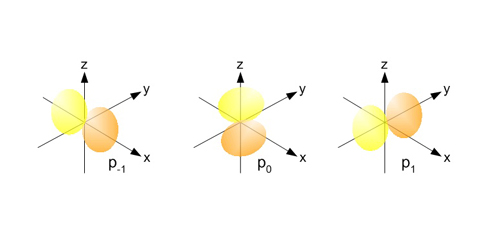Figure 2: The "figure eight" p orbitals. image © UC Davis ChemWiki

The third type of wave function has a probability density that depends on both the distance from the nucleus and the orientation along two of the x-, y-, and z-axes in space. This leads to five separate, equivalent wave functions. When the wave functions have equivalent energy, they are given the label "degenerate." They have complex shapes in 3D-space. These wave functions are all found to have values of l = 2 and can take on five different m values. These are called d orbitals, and every energy level except the first and second has such a wave function (Figure 3).Figure 3: The d orbitals, the beginning of more complex orbital shapes. image © UC Davis ChemWiki

The fourth type is a set of orbitals with even more complexity in terms of their wave function, positions, and shape in 3D-space. These wave functions are all found to have values of l = 3 and can take on seven different m values. They, too, are all degenerate. These are called f orbitals and every energy level except the first, second, and third has such a wave function, starting with 4f and moving to 5f (Figure 4).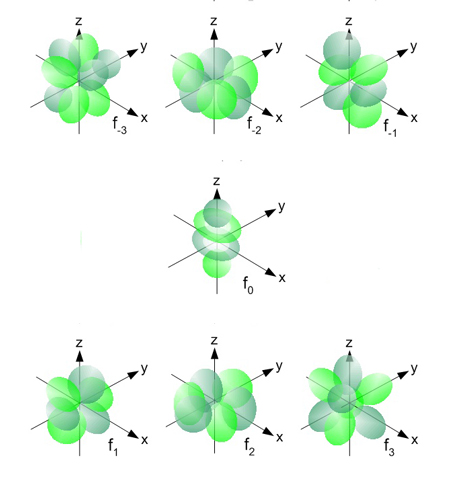Figure 4: The f orbitals, which continue the complexity of shape as seen in the d orbitals. image © UC Davis ChemWiki

To summarize, the orbitals available in the first four energy levels are as follows in Table 2:

Table 2: Orbitals associated with the first four energy levels.
n orbitals
1 1s
2 2s, 2p
3 3s, 3p, 3d
4 4s, 4p, 4d, 4f

Punto de Comprensión

The principal quantum number n

### Evidence for orbitals and the quantum model

Since orbitals are defined by very specific solutions to the Schrödinger equation, electrons must absorb very specific quantities of energy to be promoted from one energy level to another. By exposing electrons to an external source of energy, such as light, it is possible to promote the electrons from their ground state to other positions that have higher potential energies, called excited states. These ‘excited’ electrons quickly return to lower energy positions (in order to regain the stability associated with lower energies), and in doing so they release energy in specific frequencies that correspond to the energy differences between electron orbitals, or shells. Light energy is related to frequency (f) and Planck’s constant (h) in the equation E = hf.

Electrons within a hydrogen atom will absorb specific frequencies of energy that correspond to the energy gaps (ΔE) between two energy levels, thus allowing the promotion of an electron from a relatively low energy level to a relatively high energy level. Examining the absorption spectrum produced when hydrogen is irradiated, we observe a pattern of lines that supports this (Figure 5).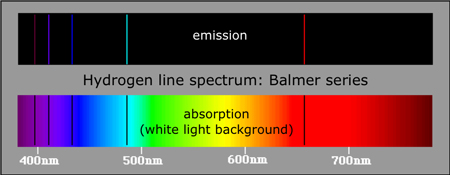Figure 5: Hydrogen's emission and absorption spectra from the Balmer series. image © Chem1 Virtual Textbook, adapted from the Online Journey through Astronomy site

Bohr proposed that electrons can transition to different positions, but only in discrete, defined steps. He thought that electrons were restricted to a specific area of space around the nucleus, much like the planets in our solar system are restricted to specific paths. The hydrogen absorption spectrum of discrete lines (rather than a continuous spectrum) shows that only very specific transitions can be made and is evidence for the quantum model.

In addition to the absorption spectrum shown above, another identical spectrum can be observed, this time with the energy being emitted rather than absorbed. In this case the electrons fall back from higher energies to lower energies rather than being promoted as in the absorption spectrum. This is called the emission spectrum of the atom and is once again made up of discrete, individual lines.

Punto de Comprensión

When energy is emitted, electrons

Each series of lines that appear in such spectra are named after their discoverers. The Lyman series are those lines in which the electrons are either promoted from, or to, the 1s orbital. In the Balmer, Paschen, Brackett, and Pfund series, electrons travel either from or to the orbitals where n = 2, 3, 4, and 5, respectively. The differing energy gaps (and therefore the differing frequencies) correspond to lines in different regions of the electromagnetic spectrum as a whole (Figure 6). Lyman lines are in the ultraviolet region, Balmer in the visible, and Paschen, Brackett, and Pfund in various parts (near and far) of the infrared.Figure 6: An energy level transition diagram for hydrogen.

In hydrogen atoms, and other single electron species such as He+ and Li2+, it is found that the energy of the orbitals is only dependent on their distance from the nucleus, with increasing energies as n increases. The lowest energy orbital is 1s, followed by 2s and 2p (that have the same energy), and 3s, 3p, and 3d (that have the same energy) etc. As such, the ground state (lowest energy state) for a hydrogen atom with only one electron is with the electron residing in the 1s orbital. The differences in energies (ΔE) required to promote electrons from one level to another can be determined by using one version of the Rydberg Formula, where RH is a constant for hydrogen and ni and nf are the initial and final energy levels respectively.Equation 3: The Rydberg Formula (created with CodeCogs online tool.)

Since other single electron species such as He+ and Li2+ contain more protons than the hydrogen atom, the electron experiences a greater attraction from the nucleus. As such, differing, larger amounts of energy are required for promotion of electrons in these species and a modified Rydberg constant is required in the calculation of ΔE for single electron species other than the hydrogen atom.

Punto de Comprensión

In atoms that have only one electron, the energy of the orbitals is dependent on the

## The more complex case of many-electron atoms

The hydrogen atom is the simplest case to study since its single electron is both uninfluenced by other electrons and does not influence any other electrons. In this case, the wave function is relatively easy to compute. However, in more complex atoms the calculations are not so easy. For example, when two electrons are present, as in helium, things become considerably more complex. Rather than there being just one simple potential energy attraction between the nucleus and the single electron in a hydrogen atom, in helium there are now three potential energy terms to consider: the attractive forces between the nucleus and each electron, and the repulsion between the two electrons. The addition of even more electrons leads to the equation and the calculations becoming increasingly complex.

The complexity of the Schrödinger equation under these many electron circumstances means that it has to be solved by a series of approximations rather than directly. One method is to effectively apply the single electron system over and over again to produce what amounts to an approximate answer. Although not entirely correct, such approximations prove to be very workable and produce reasonable answers to the multi-electron problem.

The approximation works well enough to once again produce Ψ2 wave functions that predict the electron density in space of multi-electron atoms. The orbitals in the single electron situation and those in the multi-electron situation are still defined by the same set of restricted quantum numbers, but one difference arises regarding the specific energies of those orbitals. Previously, the energy depended only on the distance from the nucleus (that is, the energy n in multi-electron systems is found to be dependent on the value of l). This complicates the idea that relates electron energy simply to distance from the nucleus (i.e., orbital energy follows the pattern 1s < (2s = 2p) < (3s = 3p = 3d), etc.). Instead, it produces the following sequence of relative energies for the orbitals.

1s < 2s < 2p < 3s < 3p < 4s < 3d < 4p < 5s < 4d < 5p, etc.

Note the insertion of 4s and 5s earlier in the sequence than expected. This order is reflected on the periodic table. When reaching the end of period three on the periodic table (element 18, argon), we will have filled the 1s, 2s, 2p, 3s, and 3p orbitals giving a total (as expected) of 18 electrons. One would perhaps expect the next orbitals to be filled to be the 3d, since after all, they are also in the third energy level. However, the next elements on the periodic table, potassium and calcium (elements 19 and 20, respectively), fill their 4s orbitals before their 3d. Moving further along the fourth period to element 21, scandium, we find that the 3d subshell now begins to populate. When that subshell is full at element 30, zinc, we then fill the 4p subshell beginning with element 31, gallium. In short, the periodic table is ordered in such a way that it reflects the sequence of relative energies of orbitals given above.

### Filling orbitals with many electrons

As seen previously, the concept of electron spin introduced the need for the fourth and final quantum number, the spin quantum number, s. Its introduction ensures that each electron has a unique set of quantum numbers according to the Pauli Exclusion Principle. With there being only two values for s (+½ or -½), it follows that each orbital may only hold a maximum of two electrons.

The notation used to describe the electronic configuration of an atom involves the use of a superscript to denote the number of electrons in any given orbital. For example, in the case of beryllium that has a total of four electrons, 1s2 2s2 shows that both the 1s and the 2s orbitals are each filled with two electrons.

### Hund's Rule - filling degenerate orbitals

When identifying which orbitals the electrons in an atom occupy, we generally follow the principle referred to as the Aufbau principle, where lower energy orbitals are filled first. So, for example, 1s is filled before 2s, and 2s before 2p, etc. But what about degenerate orbitals, those that have the same energy, such as the three orbitals that exist in the 2p sub-level?

Hund’s rule states that when electrons are placed into any set of degenerate orbitals, one electron is placed into each orbital before any spin pairing takes place. This means, for example, if we consider three electrons being placed into the degenerate 2p orbitals, one would see an electronic configuration of 2px1 2py1 2pz1 (recall the p orbitals are oriented along x, y, and z axes, respectively) before we see any pairing. The fourth electron to enter the 2p sub-shell would create the need for an unavoidable pairing, hence 2px2 2py1 2pz1 (Figure 7).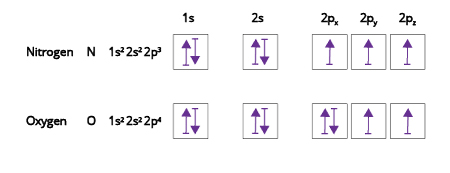Figure 7: An illustration of Hund's Rule showing the placement of electrons in various orbitals of nitrogen (N) and oxygen (O).

Now that we have a sense of electron configuration, their energies, and their most probable positions within atoms, the next step is to describe the behavior of electrons when atoms interact with one another. Electron interaction forms the basis of a key chemical process called chemical bonding. And understanding the behavior of electrons provides us with a better understanding of the chemical behavior of the elements and their compounds.

### Resumen

Our Atomic Theory series continues, exploring the quantum model of the atom in greater detail. This module takes a closer look at the Schrödinger equation that defines the energies and probable positions of electrons within atoms. Using the hydrogen atom as an example, the module explains how orbitals can be described by type of wave function. Evidence for orbitals and the quantum model is provided by the absorption and emission spectra of hydrogen. Other concepts include multi-electron atoms, the Aufbau Principle, and Hund’s Rule.

### Conceptos Clave

• The wave-particle nature of electrons means that their position and momentum cannot be described in simple physical terms but must be described by wave functions.

• The Schrödinger equation describes how the wave function of a wave-particle changes with time in a similar fashion to the way Newton’s second law describes the motion of a classical particle. The equation allows the calculation of each of the three quantum numbers related to individual atomic orbitals (principal, azimuthal, and magnetic).

• The Heisenberg uncertainty principle establishes that an electron’s position and momentum cannot be precisely known together; instead we can only calculate statistical likelihood of an electron’s location.

• The discovery of electron spin defines a fourth quantum number independent of the electron orbital but unique to an electron. The Pauli exclusion principle states that no two electrons with the same spin can occupy the same orbital.

• Quantum numbers, when taken as a set of four (principal, azimuthal, magnetic and spin) describe acceptable solutions to the Schrödinger equation, and as such, describe the most probable positions of electrons within atoms.

• Orbitals can be thought of as the three dimensional areas of space, defined by the quantum numbers, that describe the most probable position and energy of an electron within an atom.

• ##### NGSS
• HS-C1.4, HS-C4.4, HS-PS1.A2, HS-PS2.B3
• ##### Referencias
• Heisenberg, W. (1927). Über den anschaulichen Inhalt der quantentheoretischen Kinematik und Mechanik. Zeitschrift für Physik, 43(3–4), 172-198.

• Pauli, W. (1925). Ueber den Einfluss der Geschwindigkeitsabhaengigkeit der Elektronenmasse auf den Zeeman-Effekt. Zeitschrift für Physik 31(1), 373-385.
• Pauli, W. (1925). Ueber den Zusammenhang des Abschlusses der Elektronengruppen im Atom mit der Komplexstruktur der Spektren. Zeitschrift für Physik 31(1), 765-783.
• Pauli, W. (1946). Remarks on the history of the Exclusion Principle. Science, New Series, 103(2669), 213-215.
• Schrödinger, E. (1926). Quantisierung als Eigenwertproblem. Annalen der Physik, 384(4), 361-376.
• Stoner, E.C. (1924). The distribution of electrons among atomic energy levels. The London, Edinburgh and Dublin Philosophical Magazine (6th series), 48, 719-736.

Adrian Dingle, B.Sc., Anthony Carpi, Ph.D. “Atomic Theory IV” Visionlearning Vol. CHE-3 (7), 2016.

Top